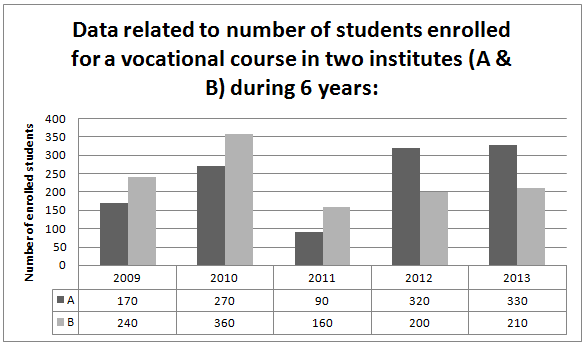# Cairn Reasoning Question

DIRECTIONS for the questions 1 to 5: Study the following information to answer the given question.
Eight friends, J, K, L, M, S, T, U and V are seated in a straight line, but not necessarily in the same order. Some are them are facing north while some face south.
• K sits second from the end of the line. U sits third to the left of K.
• T faces south. V sits fourth to the left of T. T is not an immediate neighbor of U. T does not sit at any of the extreme ends of the line.
• Both the immediate neighbors of J face north. J does not sit at any of the extreme ends of the line.
• J faces a direction opposite to that of V. (i.e. If V faces north then J faces south and vice-versa.)
• L sits second to the left of J.
• Immediate neighbors of K face opposite directions (i.e. if one neighbor faces north then the other faces south and vice-versa.)
• Persons sitting at extreme ends face opposite directions (i.e. if one person faces north then the other person faces south and vice-versa.)
• As many people sit between U and J as between U and S.
• Immediate neighbors of U face same directions (i.e. if one neighbor faces north then the other also faces north and vice-versa).
1. Which of the following is true based on the given arrangement?
1. V sits at one of the extreme ends of the line,
2. L faces north,
3. None of the given options is true
4. Only three people face north
5. S and U face the same directions
M(n) K(s) T(s) S(s) U(n) J(s) V(n) L(s)
n = north and s = south
Only three people face north is true option on the given arrangement.
2. Who amongst the following faces north?
1. L
2. M
3. J
4. K
5. S
M(n) K(s) T(s) S(s) U(n) J(s) V(n) L(s)
n = north and s = south
From the options, M faces north.
3. Who amongst the following sits third to the left of S?
1. K
2. J
3. V
4. L
5. No one as less than three people sits to the left of S.
M(n) K(s) T(s) S(s) U(n) J(s) V(n) L(s)
n = north and s = south
V sits third to the left of S.
4. How many people sit exactly between T and U?
1. Four
2. Three
3. Two
4. More than four
5. One
M(n) K(s) T(s) S(s) U(n) J(s) V(n) L(s)
n = north and s = south
One people sit exactly between T and U.
5. Who among the following represent the persons sitting at extreme ends of the line?
1. M, V
2. M, S
3. L, V
4. M, L
5. S, V
M(n) K(s) T(s) S(s) U(n) J(s) V(n) L(s)
n = north and s = south
M, L represent the persons sitting at extreme ends of the line.
DIRECTIONS for question 6 to 8: Refer to the bar graph and answer the given question.1. What is the difference between average number of students enrolled in institute A in 2009 and 2010 and that in institute B in 2011 and 2012?
1. 20
2. 40
3. 30
4. 10
5. 60
A = 440/2 = 220 and B = 360/2 = 180
Difference = 220 – 180 = 40
2. If the number of enrolled students in institute A in 2013 is 25% less than that in 2014, how many students were enrolled in institute A in 2014?
1. 450
2. 480
3. 440
4. 500
5. 490
Let students enrolled in 2014 In institute A be ‘x’ 85% of x = 330 ⇒ x = 440
3. In 2009, 65% of students enrolled in institute B were male. If the respective ratio between number of male students enrolled in institutes A and B in 2009 was 3: 4, what was the number of male students enrolled in institute A in the same year?
1. 111
2. 117
3. 123
4. 114
5. 105
Male students of B = 65% * 240 =156
Total students male = 4/7 x = 156 ⇒ x = 273
Male students of A = 273 -156= 117
DIRECTIONS for questions 9 to 10: Read the following information and answer the given question:
Vansh starts walking from Point E and walks 25m towards the north. He then takes a right turn and walks for 15m. He makes a left turn and stops at Point M after walking for 20m.
Point K is 30m to the west of Point M. Point K is 45m to the north of Point J. Point J is 10m to the east of Point L.
1. How far and in which direction is Point E with respect to Point L?
1. 30m towards West
2. 25m towards East
3. 20m towards East
4. 20m towards West
5. 25m towards West